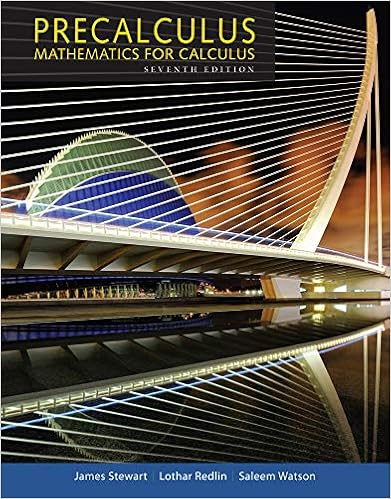# Q3 consider the simple linear regression model y i x

• Test Prep
• 4
• 100% (1) 1 out of 1 people found this document helpful

This preview shows page 1 - 3 out of 4 pages.

##### We have textbook solutions for you!
The document you are viewing contains questions related to this textbook.The document you are viewing contains questions related to this textbook.
Chapter 1 / Exercise 14
Precalculus: Mathematics for Calculus
Redlin/StewartExpert Verified
Q3.Consider the simple linear regression modelyi|xiind∼ N(β0+β1xi, σ2),i= 1, . . . , n.1
##### We have textbook solutions for you!
The document you are viewing contains questions related to this textbook.The document you are viewing contains questions related to this textbook.
Chapter 1 / Exercise 14
Precalculus: Mathematics for Calculus
Redlin/StewartExpert Verified
Midterm 1: Practice Question Solutionsa. Write this model is matrix form,y∼ N(Xβ, σ2I), and specify the values and dimen-sions ofy,X, andβ.b. Show that the MLEsˆβ0andˆβ1have covariancecov(ˆβ0,ˆβ1) =σ2¯xSxx.Q4.Consider the multiple linear regression modely∼ N(Xβ, σ2I)⇐⇒yi|xiind∼ N(∑pj=1xijβj, σ2),i= 1, . . . , n.Suppose that the matrixX=bracketleftbiggx11···x1p......xn1···xnpbracketrightbigghas the property thatnk=1xkixkj= 0 for anyinegationslash=j. Show that the MLEˆβconsists of independent random variables.Q5.Consider the multiple linear regression modely∼ N(Xβ, σ2I)⇐⇒yi|xiind∼ N(∑pj=1xijβj, σ2),i= 1, . . . , n.a. Let ˆy=Xˆβande=yˆy. Show thatni=1ˆyiei= ˆye= 0.b. Show thatee=yyˆβXXˆβ.c. Show that for any vectora= (a1, . . . , ap),aXe= 0.d. Show that1σ2(ˆββ)XX(ˆββ)χ2(p).Q6.Consider the multiple linear regression modely∼ N(Xβ, σ2I)⇐⇒yi|xiind∼ N(∑pj=1xijβj, σ2),i= 1, . . . , n.a. Suppose thatn= 50 and,but unfortunately the values of the elements in the lower corner of (XX)1have beenlost. Can you still findˆβ? If so, please calculate it. Otherwise, explain why not.b. In addition to the data above, suppose thatni=1(yixiˆβ)2= 2.
•••